# Multiple Linear Regression

In the last two articles, we explored the concept of Simple Linear Regression Model (i.e., regression involving two variables). Although, the practical situations demand much more complexity, for almost all the situations when we apply this concept in real life, we have numerous variables (which might or might not affect the outcome variable significantly). Let’s learn how to build and optimize our Multiple Linear regression model (model with more than one explanatory variables).

Example: Lets consider the following dataset using which, we intend to explain the price of the flat (Y) using the following explanatory variables: Number of Bedrooms, Number of Bathrooms, square feet of the living area, the floor on which it is located and the Grading of the flat (given by the customers earlier).

Link to the dataset: https://drive.google.com/file/d/1mxVohtxi8Qe0sPehTiEa2_vFjQxXTGOK/view?usp=sharing
source: Kaggle

# Before fitting the Multiple Regression Model

We might be eager to jump right into the model fitting and the predictions, but hold on. We first have to study the patterns and relations in out dataset. Hence, we make a correlations matrix for all the variables involved, to study their correlations amongst one another.

R code:
> plot(data)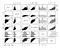Image by author

Clearly, there are a lot of linear relations as seen from the scatterplots of the variables. Our main objective is- to minimize the inter-correlations between the explanatory variables and to maximize the correlations between the explanatory and outcome variable.

Here, the Price of the flat seems to the linearly related to all the other variables, as seem from the first row of the plot. Although there are some relations amongst the explanatory variables like number of bathrooms and the square feet of the living room, multicollinearity should be avoided in an efficient model. The tests for multicollinearity are discussed later.

# Fitting a Multiple Regression Model to explain Y-Price of the Flat

Lets make use of the R software to understand the fit the model and understand the key factors that need to be studies in order to optimize our Model.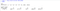Output window of the R code

Now, we see that the intercepts are estimated and their values for each of the corresponding explanatory variable is mentioned above. Hence, the model becomes,

Note: We witness that the coefficients are not in the similar scale. We can improve the model better, by apply feature scaling, where we scale the variables with large differences in the observations to get a better and more efficient model.

By running the code:
> summary(model)

In order to begin the process of optimization for the model, we look at the p-value of the F-test for the significance of the multiple regression. Here, we get, p-value < 2.2e^-16 < 0.05(alpha). Hence, we reject H0 at 5% level of significance and conclude that at least one of the variables is being able to explain the output variable significantly, and that, regression is valid.

Also, the term “R² ” is used very frequently in the modelling phase. Here, we get R² value equal to 54.56%. Now, R² always increases with the rise in the number of variables (but we know, we don’t wish to stuff our model with variables). Therefore, Adjusted R² was introduced, this value balances out the rise in R² due to variables with the actual increase in efficiency of the model. Here, adjusted R² is equal to 54.55%.

# Optimization of the Model

We might be tempted to keep as many variables as our dataset provides, but we have to remember, a complex model only makes our model less efficient and reduces its predictive capacities.

Hence, it is important to first study the correlations between the variables, study their significance, make sure we have the least correlation between the explanatory variables in themselves and significant correlation with the outcome variable, as done earlier.

Now, major optimization of the model happens while removing the unnecessary variables from the model. Surprisingly, the variables from the output snapshot above, are all significant in explaining the price of the flat (this is because, the p-value of all the estimates-slope and intercept- is less than 0.05).

Not only this, for the advanced forms of model fitting, we have to take care of heteroscedasticity. Its when the residuals do not have a constant variance. The opposite of this case is called Homoscedasticity. Plot: Plot 3 of Diagnostic Plots. Test: Spearman’s rank correlation test. Some advanced tests also include, Breush-Pagan test and the NCV test. If we find heteroscedasticity, we can use the Box-Cox transformation to improve the variables and increase homoscedasticity.

Test for multicollinearity: VIF (Variance Inflation Factor) and Farrar Glauber.

Test for Autocorrelations: Autocorrelation is when the residuals of the model are correlated. Now, one of the assumptions of building a multiple linear regression is to have no correlation between the residuals. Hence, this causes errors in predictions and has to be taken care of. Tests: Runs test.

# Diagnostic Plots

R code:
> plot(model)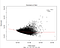Residual vs Fitted Plot, Image by author

In the above plot, we see that the scatter points are clumped together, this is not a good sign of the linearity assumptions. Ideally, the scatter points should be random and the red line should be straight and horizontal.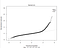Normal QQ plot, Image by author

For the QQ plot, the points should seem to follow a straight line, whereas in this case, they tend to tapper off from the straight black dotted line. Hence, we can say that, the residuals do not exactly satisfy the assumptions of normality of the residuals of a linear regression model.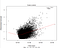Scale-Location Plot, Image by author

This plot is used to observe the trend in the homogeneity of the variance. This plot is used for the analysis of Homoscedasticity. Random points and a straight line is a good indicator of homoscedasticity. But clearly, this is not the case for the model above.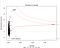Residual vs Leverage plot, Image by author

This plot is used to identify the outliers in the model. It highlights those cases or observations, which are the outliers for the model and hence affect the estimates significantly, to tip them with their behavior (which disturbs the model and skews from what is real). Here, we can see that observations 3915, 15871 and 7253 are marked as outliers.

# Limitations of the Analysis

This model is built from a random dataset available on Kaggle. It doesn’t take into account the scaling of the variables due to which our model contains large coefficient values. There are numerous outliers in the dataset, which make this inefficient.

In the later articles, we would be studying the various tests discussed in this article. In addition, we would look at the various ways to deal with outliers and how improve our model with various techniques Outlier Analysis.

Happy Learning!

## Nerd For Tech

From Confusion to Clarification

## Nerd For Tech

NFT is an Educational Media House. Our mission is to bring the invaluable knowledge and experiences of experts from all over the world to the novice. To know more about us, visit https://www.nerdfortech.org/. Don’t forget to check out Ask-NFT, a mentorship ecosystem we’ve started## Nerd For Tech

NFT is an Educational Media House. Our mission is to bring the invaluable knowledge and experiences of experts from all over the world to the novice. To know more about us, visit https://www.nerdfortech.org/. Don’t forget to check out Ask-NFT, a mentorship ecosystem we’ve started

## More From Medium

Medium is an open platform where 170 million readers come to find insightful and dynamic thinking. Here, expert and undiscovered voices alike dive into the heart of any topic and bring new ideas to the surface. Learn more

Follow the writers, publications, and topics that matter to you, and you’ll see them on your homepage and in your inbox. Explore

If you have a story to tell, knowledge to share, or a perspective to offer — welcome home. It’s easy and free to post your thinking on any topic. Write on Medium